# ICSE Worksheet for chapter-6 Triangles class 9

## Find ICSE Worksheet for chapter-6 Triangles class 9

CLASS-9

BOARD: ICSE

Mathematic Worksheet - 6

TOPIC: Triangles

For other ICSE Worksheet for class 9 Mathematic check out main page of Physics Wallah.

SUMMARY

#### SIMILAR TRIANGLE

1. Two triangle ABC and DEF are said to be similar if their
1. Corresponding Angles are equal.
2. I.e. A= F
And,
3. Corresponding Sides are proportional.
1. (AAA Similarity)If two triangles are equiangular, then they are similar.
2. (SSS Similarity)If the corresponding sides of two triangles are proportional, then they are similar.
3. (SAS Similarity)If in two triangles ,one pair of corresponding sides are proportional and the included angles are equal then the two triangle are similar

#### THALES THEOREM (BASIC PROPORTIONALITY THEOREM)

Statement: if a line is drawn parallel to one side of a triangle to intersect the other two sides in distinct points then the other two sides are divided in the same ratio.

#### AREA OF SIMILAR TRIANGE

Two triangles ABD and PQR such that ΔABC~ΔPQR

(ar(ABC))/(ar(PQR))=(AB/PQ)2=(BC/QR)2=(CA/RP)2

#### OBJECTIVE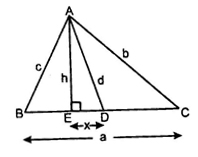1. In the following figure AE BC,D is the midpoint of BC then x is equal to:
1. 1/a (b2-d2-a2/4)
2. (h+d)/3
3. (c+d-h)/2
4. (a2+b2+d2-c2)/2
2. Two triangles ABC and PQR are similar, If BC:CA:AB=1:2:3 then QR/PR is:
1. 2/3
2. 1/2
3. 1/ √2
4. 2/3
3. In a triangle ABC if an angle B=90o and D is the point in BC such that BD=2DC then
4. AC2=AB2+5BD2
4. P and Q are the mid points of the sides AB and BC respectively of the triangle ABC, the Right angle triangle at B then,
1. AQ2+CP2=AC2
2. AQ2+CP2= 4/5AC2
3. AQ2+CP2= 5/4AC2
4. AQ2+CP2= 3/5AC2
5. ABC is a right angled triangle, right angled at A.A circle is inscribed in it. The lengths of the two sides containing the right angle are 6 cm and 8 cm, then the radius of the circle is
1. 3 cm
2. 2cm
3. 4cm
4. d) 8cm

#### SUBJECTIVE

1. Given: ∠GHE=∠DFE=90o,,,DH=8,DF=12,DG=3x-1 and DE=4x+2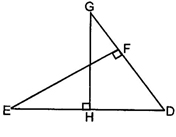Find the lengths of segments DG and DE.
2. In figure, ∠BAC=900 AD BC. Prove that AB2=BD2+AC2-CD2.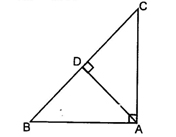3. In figure, D and E trisect BC. Prove that 8AE2=3AC​2+5AD2.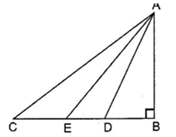4. The perpendicular AD on the base BC of a ΔABC meets BC at D so that 2DB=3CD.Prove that 5AB2=5AC2 +BC2.
5. D, E, F are the mid points of the side AB, BC, and CA respectively of ΔABC. Findar(ΔDEF)/ar(ΔABC)
6. In figure M is the midpoint of side CD, of a parallelogram ABCD. The line BM is drawn intersecting AC at L and AD produced at E. Prove that EL=2BL.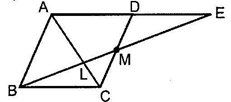7. If the area of two similar triangles are in the ratio 25:64 write the ratio of their corresponding sides.
8. 8) Prove that the ratio of the areas of two similar triangles is equal to the square of the ratio of their corresponding sides.

Using the above do the following

AD is an altitude of an equilateral triangle ABC. On AD, as base another equilateral triangle ADE is constructed.

Prove that area (ΔADE): area (ΔABC)=3:4

### Solutions: to worksheet-6 Topic-Triangles

1. a
2. b
3. b
4. c
5. b

#### Subjective:

1)20 units and 30 units

5) 1:4

7) 5:8